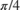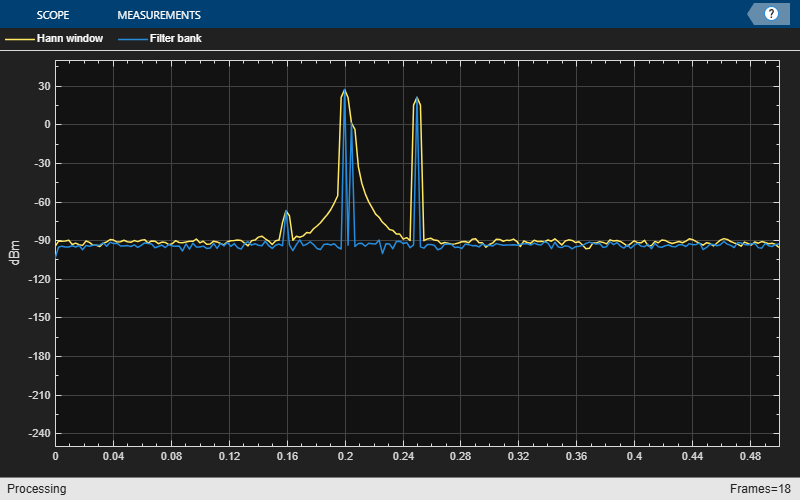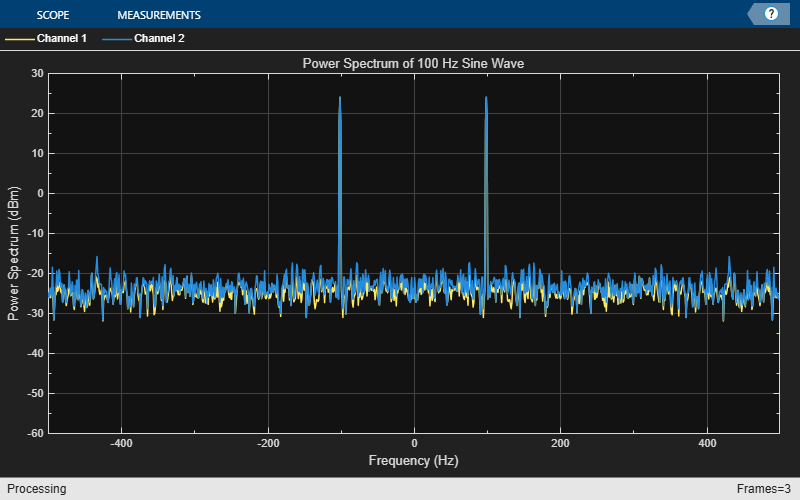# dsp.SpectrumEstimator

Estimate power spectrum or power density spectrum

## Description

The dsp.SpectrumEstimator System object™ computes the power spectrum or the power density spectrum of a signal using the Welch algorithm or the filter bank approach.

When you choose the Welch method, the object computes the averaged modified periodograms to compute the spectral estimate. When you choose the filter bank approach, an analysis filter bank splits the broadband input signal into multiple narrow subbands. The object computes the power in each narrow frequency band, and the computed value is the spectral estimate over the respective frequency band. For signals with relatively small FFT lengths, the filter bank approach produces a spectral estimate with a higher resolution, a more accurate noise floor, and peaks more precise than the Welch method, with low or no spectral leakage. These advantages come at the expense of increased computation and slower tracking.

The spectrum can be expressed in watts or in decibels. This object can also estimate the max-hold and min-hold spectra of the signal.

To estimate the power density spectrum:

1. Create the dsp.SpectrumEstimator object and set its properties.

2. Call the object with arguments, as if it were a function.

## Creation

### Syntax

SE = dsp.SpectrumEstimator
SE = dsp.SpectrumEstimator(Name,Value)

### Description

SE = dsp.SpectrumEstimator returns a System object, SE, that computes the frequency power spectrum or the power density spectrum of real or complex signals. This System object uses the Welch’s averaged modified periodogram method or the filter bank-based spectral estimation method. 

example

SE = dsp.SpectrumEstimator(Name,Value) returns a dsp.SpectrumEstimator System object with each specified property name set to the specified value. Unspecified properties have default values.

## Properties

expand all

Unless otherwise indicated, properties are nontunable, which means you cannot change their values after calling the object. Objects lock when you call them, and the release function unlocks them.

If a property is tunable, you can change its value at any time.

Spectrum type, specified as either 'Power' or 'Power density'. When the spectrum type is 'Power', the power density spectrum is scaled by the equivalent noise bandwidth of the window (in Hz).

Tunable: Yes

Source of the FFT length value, specified as either 'Auto' or 'Property'. If you set this property to 'Auto', the spectrum estimator sets the FFT length to the input frame size. If you set this property to 'Property', then you specify the number of FFT points using the FFTLength property.

Specify the length of the FFT that the spectrum estimator uses to compute spectral estimates as a positive integer.

#### Dependencies

This property applies when you set the FFTLengthSource property to 'Property'.

Data Types: double

Specify the spectral estimation method:

• 'Welch' — The object uses Welch's averaged modified periodograms method.

• 'Filter bank' — An analysis filter bank splits the broadband input signal into multiple narrow subbands. The object computes the power in each narrow frequency band, and the computed value is the spectral estimate over the respective frequency band.

Specify a window function for the spectral estimator as one of 'Rectangular', 'Chebyshev', 'Flat Top', 'Hamming', 'Hann', or 'Kaiser'.

#### Dependencies

This property applies when you set Method to 'Welch'.

Specify the number of filter coefficients, or taps, for each frequency band. This value corresponds to the number of filter coefficients per polyphase branch. The total number of filter coefficients is given by NumTapsPerBand × FFTLength.

#### Dependencies

This property applies when you set Method to 'Filter bank'.

Data Types: single | double | int8 | int16 | int32 | int64 | uint8 | uint16 | uint32 | uint64

Specify the frequency range of the spectrum estimator as one of 'twosided', 'onesided', or 'centered'.

If you set the FrequencyRange to 'onesided', then the spectrum estimator computes the one-sided spectrum of a real input signal. When the FFT length, NFFT, is even, the spectrum estimate has length (NFFT/2) + 1 and is computed over the frequency range [0, SampleRate/2], where SampleRate is the sample rate of the input signal. When NFFT is odd, the spectrum estimate has length (NFFT + 1)/2 and is computed over the frequency range [0, SampleRate/2).

If you set the FrequencyRange to 'twosided', then the spectrum estimator computes the two-sided spectrum of a complex or real input signal. The length of the spectrum estimate is equal to the FFT length. The spectrum estimate is computed over the frequency range [0, SampleRate), where SampleRate is the sample rate of the input signal.

If you set the FrequencyRange to 'centered', then the spectrum estimator computes the centered two-sided spectrum of a complex or real input signal. The length of the spectrum estimate is equal to the FFT length. The spectrum estimate is computed over the frequency range (-SampleRate/2, SampleRate/2] when the FFT length is even and (-SampleRate/2, SampleRate/2) when the FFT length is odd.

Specify the units used to measure power as one of 'Watts', 'dBW', or 'dBm'.

Specify the averaging method as 'Running' or 'Exponential'. In the running averaging method, the object computes an equally weighted average of a specified number of spectrum estimates defined by the SpectralAverages property. In the exponential method, the object computes the average over samples weighted by an exponentially decaying forgetting factor.

Number of spectral averages, specified as a positive integer. The spectrum estimator computes the current power spectrum or power density spectrum estimate by averaging the last N estimates. N is the number of spectral averages defined in the SpectralAverages property.

#### Dependencies

This property applies when you set AveragingMethod to 'Running'.

Data Types: double

Specify the exponential weighting forgetting factor as a scalar value greater than zero and smaller than or equal to one.

Tunable: Yes

#### Dependencies

This property applies when you set AveragingMethod to 'Exponential'.

Data Types: single | double

Specify the load that the spectrum estimator uses as a reference to compute power values as a real, positive scalar in ohms.

Data Types: single | double

Specify the side lobe attenuation of the window as a real, positive scalar, in decibels (dB).

#### Dependencies

This property applies when you set Method to 'Welch' and Window to 'Chebyshev' or 'Kaiser'.

Data Types: double

Set this property to true so that the spectrum estimator computes and outputs the max-hold spectrum of each input channel. The max-hold spectrum is computed by keeping, at each frequency bin, the maximum value of all the power spectrum estimates.

Set this property to true so that the spectrum estimator computes and outputs the min-hold spectrum of each input channel. The min-hold spectrum is computed by keeping, at each frequency bin, the minimum value of all the power spectrum estimates.

Sample rate of the input in Hz, specified as a finite numeric scalar. The sample rate is the rate at which the signal is sampled in time.

Data Types: single | double

## Usage

### Syntax

pxx = SE(x)
[pxx,pmax] = SE(x)
[pxx,pmin] = SE(x)
[pxx,pmax,pmin] = SE(x)

### Description

example

pxx = SE(x) computes the power spectrum or power-density spectrum, pxx, of the input signal, x. The System object treats the columns of x as independent channels.

example

[pxx,pmax] = SE(x) also computes the max-hold frequency spectrum, pmax, of x. To determine the max-hold spectrum, the method keeps the maximum of all the power spectrum estimates computed at each frequency bin. Set OutputMaxHoldSpectrum to true to obtain the max-hold spectrum.
[pxx,pmin] = SE(x) also computes the min-hold frequency spectrum, pmin, of x. To determine the min-hold spectrum, the method keeps the minimum of all the power spectrum estimates computed at each frequency bin. Set OutputMinHoldSpectrum to true to obtain the min-hold spectrum.
[pxx,pmax,pmin] = SE(x) computes the power spectrum or power-density spectrum, the max-hold spectrum, and the min-hold spectrum of x. Set OutputMaxHoldSpectrum and OutputMinHoldSpectrum to true to obtain the max-hold and min-hold spectra.

### Input Arguments

expand all

Input signal, specified as a vector or matrix. The row length of x is the frame size or channel length. Each column of x is treated as a separate channel. The column length of x is the number of channels.

Data Types: single | double
Complex Number Support: Yes

### Output Arguments

expand all

Power or power-density spectrum estimate, returned as a vector or matrix of the same data type and complexity as the input signal, x.

When FFTLengthSource is set to:

• 'Auto' –– The size of pxx is same as the size of the input signal, x.

• 'Property' –– The size of pxx is the same as the specified FFT length.

By default, the unit of pxx is 'Watts'. You can also specify the spectrum to be in 'dBm' or 'dBW' through the PowerUnits property.

Data Types: single | double
Complex Number Support: Yes

Max-hold spectrum estimate, returned as a vector or matrix of the same size, data type, and complexity as the output signal, pxx.

Data Types: single | double
Complex Number Support: Yes

Min-hold spectrum estimate, returned as a vector or matrix of the same size, data type, and complexity as the output signal, pxx.

Data Types: single | double
Complex Number Support: Yes

## Object Functions

To use an object function, specify the System object as the first input argument. For example, to release system resources of a System object named obj, use this syntax:

release(obj)

expand all

 getFrequencyVector Vector of frequencies at which estimation is done getRBW Resolution bandwidth of spectrum
 step Run System object algorithm release Release resources and allow changes to System object property values and input characteristics reset Reset internal states of System object

## Examples

collapse all

Compute the power spectrum of a multichannel sinusoidal signal using the dsp.SpectrumEstimator System object™. You can get the vector of frequencies at which the spectrum is estimated using the getFrequencyVector function. To compute the resolution bandwidth of the estimate (RBW), use the getRBW function.

Generate a three-channel sinusoid sampled at 1 kHz. Specify sinusoidal frequencies of 100, 200, and 300 Hz. The second and third channels have their phases offset from the first byand, respectively.

sineSignal = dsp.SineWave('SamplesPerFrame',1000,'SampleRate',1000, ... 'Frequency',[100 200 300],'PhaseOffset',[0 pi/2 pi/4]); 

Estimate and plot the one-sided spectrum of the signal. Use the dsp.SpectrumEstimator object for the computation and the dsp.ArrayPlot for the plotting.

estimator = dsp.SpectrumEstimator('FrequencyRange','onesided'); plotter = dsp.ArrayPlot('PlotType','Line','YLimits',[0 0.75], ... 'YLabel','Power Spectrum (watts)','XLabel','Frequency (Hz)'); 

Step through to obtain the data streams and display the spectra of the three channels.

y = sineSignal(); pxx = estimator(y); plotter(pxx)Get the vector of frequencies at which the spectrum is estimated in Hz, using the getFrequencyVector function.

f = getFrequencyVector(estimator); 

Compute the resolution bandwidth (RBW) of the estimate using the getRBW function.

rbw = getRBW(estimator) 
rbw = 0.0015 

The resolution bandwidth of the signal power spectrum is 0.0015 Hz. This frequency is the smallest frequency that can be resolved on the spectrum.

Compare spectral estimates of sinusoids embedded in white Gaussian noise using a Hann window-based Welch method and filter bank method.

Initialization

Initialize two dsp.SpectrumEstimator objects. Specify one estimator to use the Welch-based spectral estimation technique with a Hann window. Specify the other estimator to use an analysis filter bank to perform the spectral estimation. Specify a noisy sine wave input signal with 4 sinusoids at 0.16, 0.2, 0.205, and 0.25 cycles/sample. View the spectral estimate using an array plot.

FrameSize = 420; Fs = 1; sinegen = dsp.SineWave('SampleRate',Fs,... 'SamplesPerFrame',FrameSize,... 'Frequency',[0.16 0.2 0.205 0.25],... 'Amplitude',[2e-5 1 0.05 0.5]); NoiseVar = 1e-10; numAvgs = 8; hannEstimator = dsp.SpectrumEstimator('PowerUnits','dBm',... 'Window','Hann','FrequencyRange','onesided',... 'SpectralAverages',numAvgs,'SampleRate',Fs); filterBankEstimator = dsp.SpectrumEstimator('PowerUnits','dBm',... 'Method','Filter bank','FrequencyRange','onesided',... 'SpectralAverages',numAvgs,'SampleRate',Fs); spectrumPlotter = dsp.ArrayPlot(... 'PlotType','Line','SampleIncrement',Fs/FrameSize,... 'YLimits',[-250,50],'YLabel','dBm',... 'ShowLegend',true,'ChannelNames',{'Hann window','Filter bank'}); 

Streaming

Stream the input. Compare the spectral estimates computed using the Hann window and the analysis filter bank

for i = 1:1000 x = sum(sinegen(),2) + sqrt(NoiseVar)*randn(FrameSize,1); Pse_hann = hannEstimator(x); Pfb = filterBankEstimator(x); spectrumPlotter([Pse_hann,Pfb]) endThe Hann window misses the peak at 0.205 cycles/sample. In addition, the window has a significant spectral leakage that makes the peak at 0.16 cycles/sample hard to distinguish, and the noise floor is not correct.

The filter bank estimate has a very good resolution with no spectral leakage.

Note: If you are using R2016a or an earlier release, replace each call to the object with the equivalent step syntax. For example, obj(x) becomes step(obj,x).

Generate a sine wave.

sineWave = dsp.SineWave('Frequency',100,... 'SampleRate',1000, ... 'SamplesPerFrame',1000); 

Use the spectrum estimator to compute the power spectrum and the max-hold spectrum of the sine wave. Use the Array Plot to display the spectra.

SE = dsp.SpectrumEstimator(... 'SampleRate',sineWave.SampleRate,... 'SpectrumType','Power','PowerUnits','dBm', ... 'FrequencyRange','centered',... 'OutputMaxHoldSpectrum',true); plotter = dsp.ArrayPlot('PlotType','Line',... 'XOffset',-500, ... 'YLimits',[-60 30],... 'Title','Power Spectrum of 100 Hz Sine Wave', ... 'YLabel','Power Spectrum (dBm)',... 'XLabel','Frequency (Hz)'); 

Add random noise to the sine wave. Stream in the data, and plot the power spectrum of the signal.

for ii = 1:10 x = sineWave() + 0.05*randn(1000,1); [Pxx,Pmax] = SE(x); plotter([Pxx Pmax]) end## Algorithms

expand all

 Hayes, Monson H. Statistical Digital Signal Processing and Modeling. Hoboken, NJ: John Wiley & Sons, 1996

 Kay, Steven M. Modern Spectral Estimation: Theory and Application. Englewood Cliffs, NJ: Prentice Hall, 1999

 Stoica, Petre and Randolph L. Moses. Spectral Analysis of Signals. Upper Saddle River, NJ: Prentice Hall, 2005

 Welch, P. D. “The use of fast Fourier transforms for the estimation of power spectra: A method based on time averaging over short modified periodograms,” IEEE Transactions on Audio and Electroacoustics, Vol. 15, 1967, pp. 70–73.

 Harris, F.J. Multirate Signal Processing for Communication Systems. Prentice Hall. 2004, pp. 208–209.